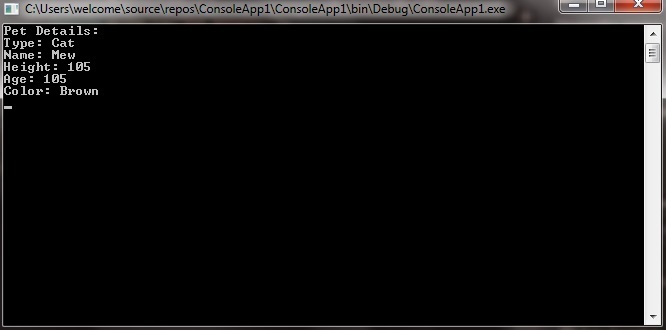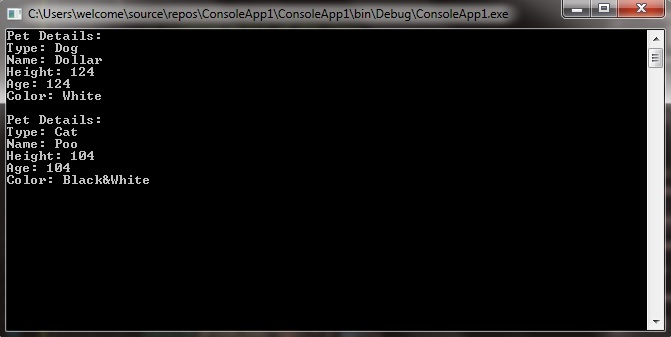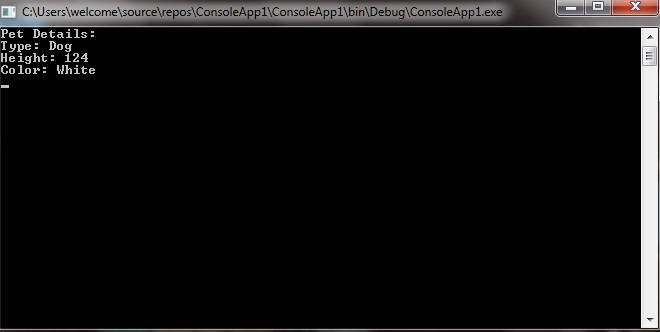# C# | Deconstructors with Tuples

A tuple is a data structure which gives you the easiest way to represent a data set which has multiple values that may/may not be related to each other. But if you are trying to retrieve multiple fields or property values from the tuple is more difficult. So, to overcome this problem deconstructor was introduced in C# 7.0. It is used to divide the variable values or tuple into parts and assigns those values to the new variables. It also used with classes and structures. Here we only discuss the working of deconstructor with tuples.

In Tuples, deconstructor is used to divide a tuple into parts and assign these parts individually into new variables. So, you can access an individual field or property value. You can deconstruct a tuple in four different ways:

1. You can deconstruct a tuple simply by explicitly declare the type of each field inside the parentheses. But you are not allowed to specify a specific type outside the parentheses even every field in the tuple is of the same type. If you try to do, then you will get an error.

Example:

 `// C# program to illustrate the concept  ` `// of deconstruction with the tuple ` `using` `System; ` `using` `System.Collections.Generic; ` `using` `System.Linq; ` `using` `System.Text; ` `using` `System.Threading.Tasks; ` ` `  `namespace` `ConsoleApp1 { ` ` `  `class` `GFG { ` ` `  `    ``// This method returns the Pet details ` `    ``public``(``string``, ``string``, ``int``, ``int``, ``string``) PetDetails(``string` `type,  ` `                     ``string` `name, ``int` `height, ``int` `age, ``string` `color) ` `    ``{ ` `        ``string` `p_type = type; ` `        ``string` `p_name = name; ` `        ``int` `p_height = height; ` `        ``int` `p_age = age; ` `        ``string` `p_color = color; ` `        ``return` `(p_type, p_name, p_height, p_height, p_color); ` `    ``} ` ` `  `    ``// Main method ` `    ``static` `void` `Main(``string``[] args) ` `    ``{ ` ` `  `        ``// Creating object of GFG class ` `        ``GFG g = ``new` `GFG(); ` ` `  `        ``// Deconstruct the given tuple ` `        ``// So that we can directly access individual fields ` `        ``// By explicitly declaring types ` `        ``(``string` `type, ``string` `name, ``int` `height, ``int` `age, ``string` `color) = g.PetDetails(``"Dog"``,  ` `                                                                ``"Dollar"``, 124, 3, ``"White"``); ` `        ``Console.WriteLine(``"Pet Details:"``); ` `        ``Console.WriteLine(``"Type: "` `+ type); ` `        ``Console.WriteLine(``"Name: "` `+ name); ` `        ``Console.WriteLine(``"Height: "` `+ height); ` `        ``Console.WriteLine(``"Age: "` `+ age); ` `        ``Console.WriteLine(``"Color: "` `+ color); ` `        ``Console.ReadLine(); ` `    ``} ` `} ` `} `

Output:2. You can deconstruct a tuple by using var keyword so that C# infers the type of each variable. You can use the var keyword in two different ways:

• You are allowed to place the var keyword outside of the parentheses.
• You are allowed to place the var keyword individually inside the parentheses with some or all variables.

Example:

 `// C# program to illustrate the concept  ` `// of deconstruction with the tuple ` `using` `System; ` `using` `System.Collections.Generic; ` `using` `System.Linq; ` `using` `System.Text; ` `using` `System.Threading.Tasks; ` ` `  `namespace` `ConsoleApp1 { ` ` `  `class` `GFG { ` ` `  `    ``// This method returns the Pet details ` `    ``public``(``string``, ``string``, ``int``, ``int``, ``string``) PetDetails(``string` `type,  ` `                     ``string` `name, ``int` `height, ``int` `age, ``string` `color) ` `    ``{ ` `        ``string` `p_type = type; ` `        ``string` `p_name = name; ` `        ``int` `p_height = height; ` `        ``int` `p_age = age; ` `        ``string` `p_color = color; ` `        ``return` `(p_type, p_name, p_height, p_height, p_color); ` `    ``} ` ` `  `    ``// Main method ` `    ``static` `void` `Main(``string``[] args) ` `    ``{ ` ` `  `        ``// Creating object of GFG class ` `        ``GFG g = ``new` `GFG(); ` ` `  `        ``// Deconstruct the given tuple ` `        ``// So that we can directly  ` `        ``// access individual fields ` `        ``// Using var keyword ` `        ``var``(type1, name1, height1, age1, color1) = g.PetDetails(``"Dog"``, ` `                                           ``"Dollar"``, 124, 3, ``"White"``); ` `        ``Console.WriteLine(``"Pet Details:"``); ` `        ``Console.WriteLine(``"Type: "` `+ type1); ` `        ``Console.WriteLine(``"Name: "` `+ name1); ` `        ``Console.WriteLine(``"Height: "` `+ height1); ` `        ``Console.WriteLine(``"Age: "` `+ age1); ` `        ``Console.WriteLine(``"Color: "` `+ color1); ` ` `  `        ``(``var` `type2, ``var` `name2, ``var` `height2, ``var` `age2, ``var` `color2) = g.PetDetails(``"Cat"``,  ` `                                                         ``"Poo"``, 104, 1, ``"Black&White"``); ` `        ``Console.WriteLine(``"\nPet Details:"``); ` `        ``Console.WriteLine(``"Type: "` `+ type2); ` `        ``Console.WriteLine(``"Name: "` `+ name2); ` `        ``Console.WriteLine(``"Height: "` `+ height2); ` `        ``Console.WriteLine(``"Age: "` `+ age2); ` `        ``Console.WriteLine(``"Color: "` `+ color2); ` `        ``Console.ReadLine(); ` `    ``} ` `} ` `} `

Output:3. You can deconstruct a tuple into a variable that are already declared. As shown in the below example:

Example:

 `// C# program to illustrate the concept  ` `// of deconstruction with the tuple ` `using` `System; ` `using` `System.Collections.Generic; ` `using` `System.Linq; ` `using` `System.Text; ` `using` `System.Threading.Tasks; ` ` `  `namespace` `ConsoleApp1 { ` ` `  `class` `GFG { ` ` `  `    ``// This method returns the Pet details ` `    ``public``(``string``, ``string``, ``int``, ``int``, ``string``) PetDetails(``string` `type,  ` `                     ``string` `name, ``int` `height, ``int` `age, ``string` `color) ` `    ``{ ` `        ``string` `p_type = type; ` `        ``string` `p_name = name; ` `        ``int` `p_height = height; ` `        ``int` `p_age = age; ` `        ``string` `p_color = color; ` `        ``return` `(p_type, p_name, p_height, p_height, p_color); ` `    ``} ` ` `  `    ``// Main method ` `    ``static` `void` `Main(``string``[] args) ` `    ``{ ` ` `  `        ``// Creating object of GFG class ` `        ``GFG g = ``new` `GFG(); ` ` `  `        ``// Declaring and initializing variables ` `        ``string` `type = ``"Cow"``; ` `        ``string` `name = ``"BooBoo"``; ` `        ``int` `height = 234; ` `        ``int` `age = 4; ` `        ``string` `color = ``"Black&white"``; ` ` `  `        ``// Deconstruct the given tuple ` `        ``// So that we can directly  ` `        ``// access individual fields ` `        ``// By declaring variables ` `        ``(type, name, height, age, color) = g.PetDetails(``"Cat"``,  ` `                                      ``"Mew"``, 105, 2, ``"Brown"``); ` `        ``Console.WriteLine(``"Pet Details:"``); ` `        ``Console.WriteLine(``"Type: "` `+ type); ` `        ``Console.WriteLine(``"Name: "` `+ name); ` `        ``Console.WriteLine(``"Height: "` `+ height); ` `        ``Console.WriteLine(``"Age: "` `+ age); ` `        ``Console.WriteLine(``"Color: "` `+ color); ` `        ``Console.ReadLine(); ` `    ``} ` `} ` `} `

Output:4. You can also use discards in deconstruction. Discards are write-only variables whose values are meant to be ignored. And discards are designated by using an underscore character (“_”) in an assignment. You are allowed to discard as many values as you want and all are represented by the single discard, _.

Syntax:

(var1, _, var3, _, var5) = method_name(var1_values, var2_values, var3_value, var4_values, var5_value);

Example:

 `// C# program to illustrate the concept ` `// of deconstruction with tuple ` `using` `System; ` `using` `System.Collections.Generic; ` `using` `System.Linq; ` `using` `System.Text; ` `using` `System.Threading.Tasks; ` ` `  `namespace` `ConsoleApp1 { ` ` `  `class` `GFG { ` ` `  `    ``// This method returns  ` `    ``// the Pet details ` `    ``public``(``string``, ``string``, ``int``, ``int``, ``string``) PetDetails(``string` `type, ` `                     ``string` `name, ``int` `height, ``int` `age, ``string` `color) ` `    ``{ ` `        ``string` `p_type = type; ` `        ``string` `p_name = name; ` `        ``int` `p_height = height; ` `        ``int` `p_age = age; ` `        ``string` `p_color = color; ` `        ``return` `(p_type, p_name, p_height, p_height, p_color); ` `    ``} ` ` `  `    ``// Main method ` `    ``static` `void` `Main(``string``[] args) ` `    ``{ ` ` `  `        ``// Creating object of GFG class ` `        ``GFG g = ``new` `GFG(); ` ` `  `        ``// Discarding field values ` `        ``// in deconstruction ` ` `  `        ``(``string` `type, _, ``int` `height, _, ``string` `color) = g.PetDetails(``"Dog"``, ` `                                                ``"Dollar"``, 124, 3, ``"White"``); ` `        ``Console.WriteLine(``"Pet Details:"``); ` `        ``Console.WriteLine(``"Type: "` `+ type); ` `        ``Console.WriteLine(``"Height: "` `+ height); ` `        ``Console.WriteLine(``"Color: "` `+ color); ` `        ``Console.ReadLine(); ` `    ``} ` `} ` `} `

Output:

``

Note: In deconstruction, you need to assign each element to a variable if you eliminate any element, then the compiler will give an error. And you are not allowed to mix declarations and assignments to existing variables on the left-hand side of a deconstruction if you eliminate any element, then the compiler will give an error.

My Personal Notes arrow_drop_upCheck out this Author's contributed articles.

If you like GeeksforGeeks and would like to contribute, you can also write an article using contribute.geeksforgeeks.org or mail your article to contribute@geeksforgeeks.org. See your article appearing on the GeeksforGeeks main page and help other Geeks.

Please Improve this article if you find anything incorrect by clicking on the "Improve Article" button below.

Article Tags :

Be the First to upvote.

Please write to us at contribute@geeksforgeeks.org to report any issue with the above content.